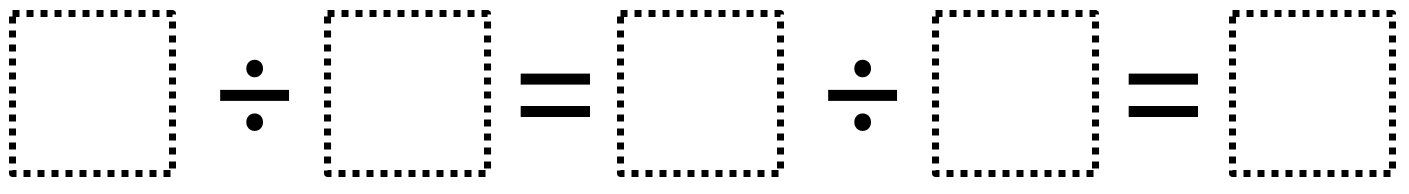Home > Grade 4 > Whole Number Division

# Whole Number Division

Directions: Using the digits 1 to 9 at most one time each, fill in the boxes to make a true statement.### Hint

What possible values can the single digit have?

### Answer

The only possible answer is 8 ÷ 4 = 6 ÷ 3 = 2

Source: Owen Kaplinsky

## Multiplying Differences

Directions: Using the digits 1 to 9 at most one time each, fill in the …

### 5 comments

1.This really puzzled my students. When we looked at the hint and then the answer, they realized there was a mistake. 8 divided by 4 is 2, not 6.

2.Thanks! What I meant to say was 8/4=2 and 6/3=2.

•That still doesn’t make a true statement, though. Is there something I’m not understanding?

•I think so. Instead of 8/4=2 and 6/3=2, you could say 8/4=6/3=2. Also, you can think of it as three parts. Each one equals the same thing. Part one is 8/4 or 2, part 2 is 6/3, or 2, and part 3 is 2.

3.Some parentheses might help